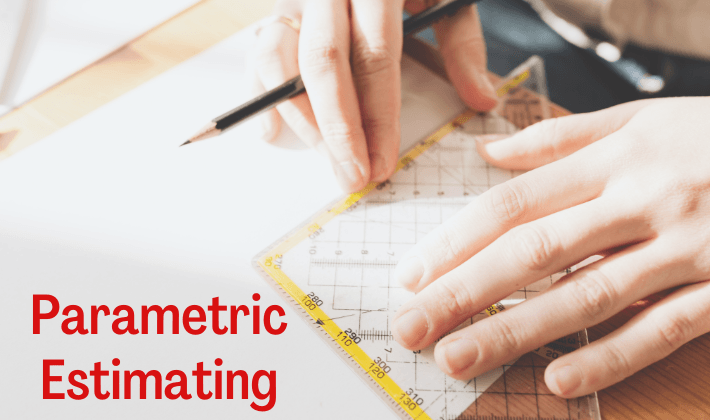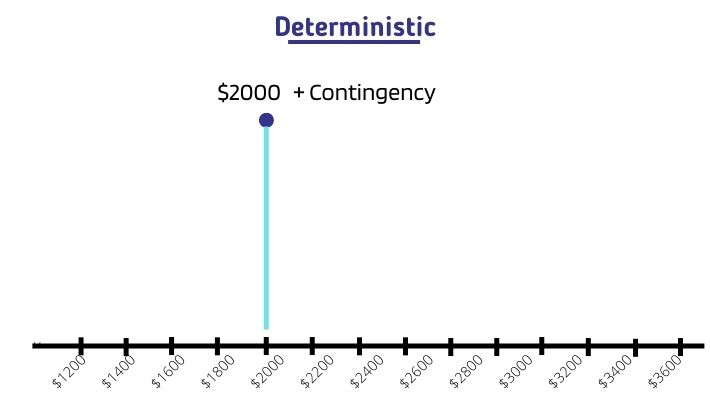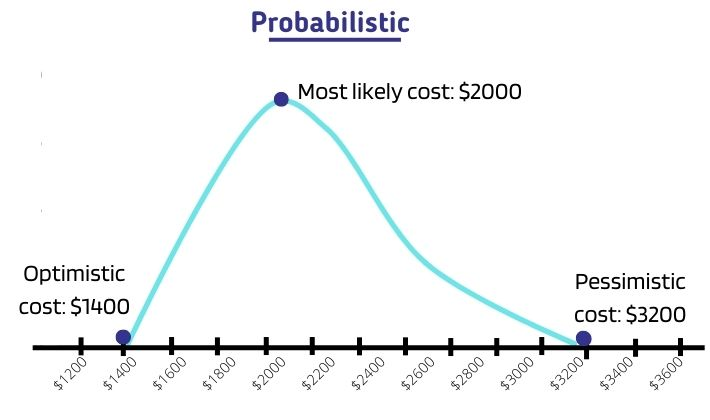Parametric estimating technique uses an algorithm or formula to calculate expected duration, cost, or resource requirements based on historical data and other project parameters. It uses statistical relationship between different project variables for estimating current values.

Parametric estimation is one of the five project management estimation methods that are described in the PMBOK Guide (A Guide to the Project Body of Knowledge). The other four methods are Expert Judgment, Analogous (also called Top-down), Bottom-up, and Three-point estimation. All these techniques are tested in the PMP (Project Management Professionals) exam that is conducted by PMI (Project Management Institute).

Unlike the other methods, Parametric method uses a data-based quantitative approach for finding the expected values. It is generally considered to be more accurate than the other methods.

Let’s get down to the brass tacks and understand the details of parametric technique. Let’s look at its generic formula and some examples to understand the concept.

## What is Parametric Estimating in Project Management?

Parametric estimation calculates expected values of duration, cost, or resources (Equipment, Material, Facility, and Human Resources) by applying a mathematical formula or algorithm on historical values. It can be used to estimate values for an entire project e.g. total cost budget or schedule. It can also be used to calculate values for a segment of project like work package or activity.

Apart from estimating time, money, and resource requirements, this technique can be used for estimating other type of project or operational variables e.g speed, volume, weight, and thrust.

The estimates derived using this technique are reliable and accurate only if there is a statistical relationship between project parameters, algorithmic model is sophisticated, and quality of underlying data is good.

## Parametric Estimating Formula

In its simplest form, parametric estimation can be done by using the following formula:

Estimated_Value = Multiplication_Factor*Current_Value

where,

• Estimated_Value is the calculated figure for the current project using parametric estimation formula
• Multiplication_Factor is a parametric factor derived from old historic data from past project e.g. duration/unit or cost/unit.
• Current_Value is the number of units of work to be done in the current project

Let’s use this formula for duration and cost estimating of a fencing project. The current project involves erecting a fence measuring 200m to make an enclosure.

Let’s assume that we had done a previous project wherein we had erected 100m of fence. That project took 10 days and \$1000 to complete.

So, by using multiplication factors for duration and cost from old project, we can estimate values for the current project.

Duration Estimation
100m fence took 10 days to complete.

So, 1m fence will take 10/100 days to complete.

Multiplication_Factor = 10/100 = 1/10

Estimated_Value (duration of current project) = (1/10)*200 = 20 days

Cost Estimation

100m fence took \$1000 to complete.

So, 1m fence will take \$(1000/100) to complete.

Multiplication_Factor = 1000/100 = 10

Estimated_Value (cost of current project) = 10*200 = \$2000

## Types of Parametric Estimation

There are two ways to apply statistical methods for getting a parametric estimate.

### 1. Deterministic Approach

In deterministic approach, a single value of estimate is calculated. Contingency reserves can be added on top of this estimate to take care of uncertainties. The above formula is a good example of deterministic approach. It can be represented by a straight line; such as the one shown in the figure below.### 2. Probabilistic Approach

In probabilistic approach, a range of values based on different probabilities is calculated. Separate contingency reserves are usually not added as range takes care of uncertainties.

The range of parametric estimates can be represented by a bell-curve (probability density curve); such as the one shown in the figure below.Probabilistic approach uses optimistic and pessimistic estimates based on a target probability and, if applicable, a multiplier to estimate standard deviations. You can refer to my other post for more information on probabilistic three point estimation.

It is very difficult to arrive at estimates without a specialized parametric estimating software.

## When to Use Parametric Model?

Parametric estimation can be used at any time during planning a project i.e., at the beginning, in the middle or towards the end. The accuracy of the calculated figures does not depend on the timing but depends upon the formula used and the quality of the underlying data. At the beginning of a project, this technique can be used for determining project cost estimate, duration, and requirement requirements for the entire project.

Parametric modelling should be done only if you can derive a statistical relationship between different variables of past and current project; such as square meter or square foot to be constructed and number of hours spent on specified units of work.

A project manager must ensure that the following conditions are met before applying this technique:

1. It is easy to identify and quantify the parameters for duration or cost estimating.
2. There is a correlation between parameters that can be used to devise a statistical relationship.
3. You have historical data for the identified parameters from a similar project.
4. The model can be scaled i.e. it doesn’t matter how many units are required to be completed.

## How to do Parametric Estimation?

You can follow the below mentioned steps to do parametric estimation:

1. Start by creating a Work Breakdown Structure (WBS). WBS represents the total scope of work. You can do parametric estimation for any component (control account, planning package, and work package) of WBS as long as conditions described in the previous section are fulfilled.
2. Decompose the work packages to activities if you want to do parametric estimation for activities.
3. Determine the tasks (WBS components or activities) that can be estimated by using parametric estimation technique. Ensure that the conditions described in the previous section are fulfilled.
4. Find historical data for the parameters that have to be estimated. You can use historical data from your previous (internal) projects or publicly available market data or data from industry and trade publications.
5. Determine correlations between current and historical parameters.
6. Create a parametric model (algorithm or formula). You can either use probabilistic and deterministic model depending on your project requirements. Make sure that the historical data can be scaled.
7. Back-test the model to check if works well for all situations.
8. Perform parametric estimating.

1. If underlying model and quality of historical data is good then this technique produces very accurate estimate.
2. It can be reused for similar projects/tasks after the parametric model is successfully created. The accuracy of the model improves as it is applied to new projects.
3. You can make manual adjustments to correct differences between historical data and current project results. Manual adjustments are a great way to correct the weaknesses in underlying model and historical data. The adjustments can also be done if the model does not fully incorporate qualitative and environmental factors.
4. It is much easier to get buy-in and approval on estimates from stakeholders because the estimates are based on mathematical logic.

1. This technique is usually more costly and time-consuming as compared to other methods. Sometimes, it takes a lot of effort and resources to create a good model and collect historical data.
2. This technique cannot be used for every segment of the project. It can be used only for those segments for which the above conditions are met.
3. It produces poor results if quality of underlying data is not good or when model is not sophisticated.
4. This technique does not account for cultural, environmental and political factors. Also, it does not consider improvement in learning, skills of personnel, and many other qualitative factors.

## Other Estimating Methods

1. Expert Judgement: It uses skills and expertise from individuals or groups that have specialized knowledge or training about the tasks that are to be estimated. Expert judgement can also be used to determine estimated values by combining other methods or to reconcile the difference between them.
2. Analogous Method: It uses values from past similar projects to figure out estimates for the current project. It is important the tasks are similar otherwise the estimation will go wrong. The analogous method is considered to be least accurate estimating method.
3. Bottom-Up Method: It decomposes the work component into smaller elements to make the estimation less complex. It then estimates each individual element separately. Finally, it aggregates the estimates of individual elements to arrive at estimate for the work component. It is more accurate and time-consuming than Analogous method.
4. Three-Point Estimating: It calculates the expected value by taking mean of three different estimates viz. optimistic, pessimistic, and most likely. It reduces the inaccuracies factoring in the uncertainties.

## Parametric vs Analogous Estimating

Both Parametric and Analogous estimating use historical data to arrive at expected values. The former uses a mathematical model to calculate expected values whereas the latter uses expert judgement on similar tasks to arrive at estimates.

Analogous estimation is also a top-down method that relies on similarities and parallels between historical tasks and current tasks.

Parametric estimating is generally more accurate than Analogous but Analogous is less complex than Parametric. You can read my other article on Parametric vs Analogous estimation to understand the difference in detail.